# Nominal GDP Vs Real GDP Krugman Section 3

• Slides: 12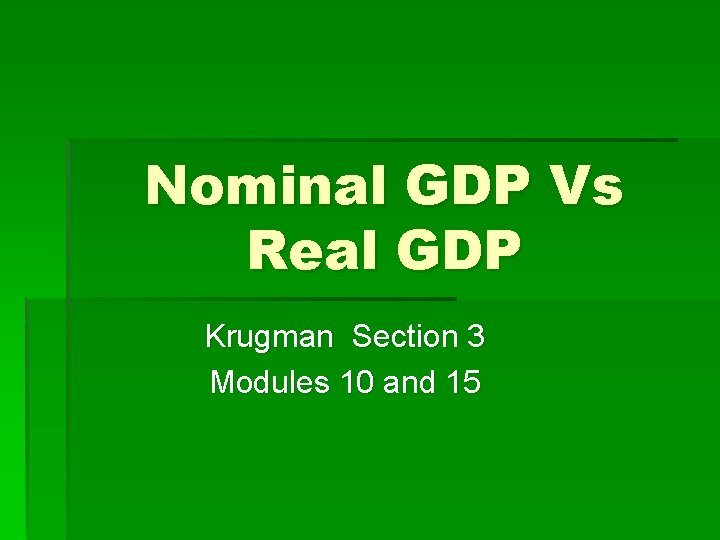Nominal GDP Vs Real GDP Krugman Section 3 Modules 10 and 15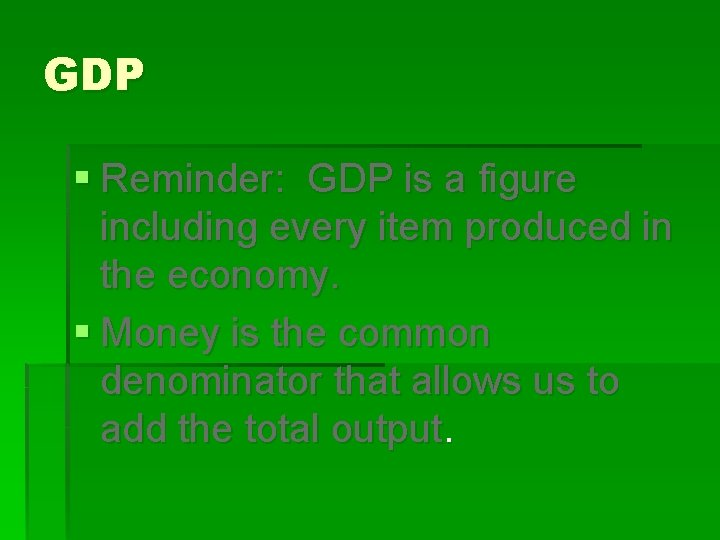GDP § Reminder: GDP is a figure including every item produced in the economy. § Money is the common denominator that allows us to add the total output.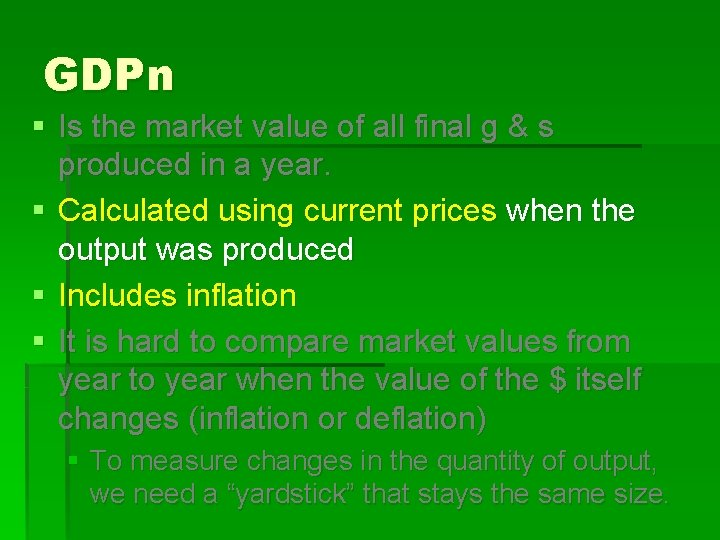GDPn § Is the market value of all final g & s produced in a year. § Calculated using current prices when the output was produced § Includes inflation § It is hard to compare market values from year to year when the value of the \$ itself changes (inflation or deflation) § To measure changes in the quantity of output, we need a “yardstick” that stays the same size.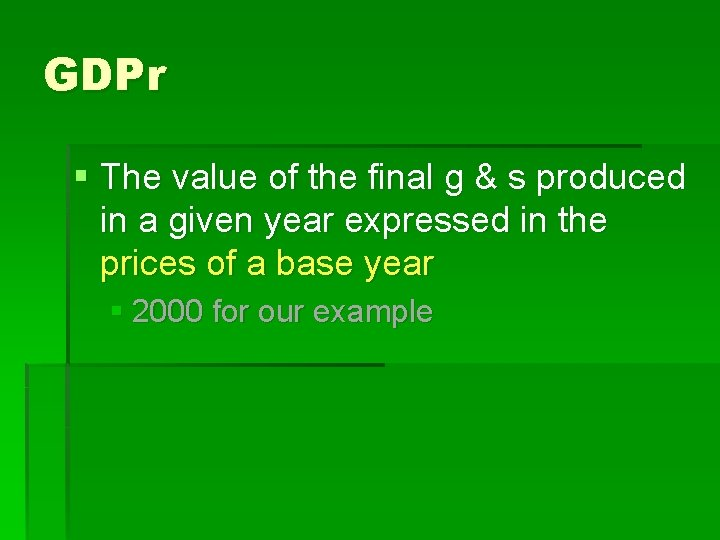GDPr § The value of the final g & s produced in a given year expressed in the prices of a base year § 2000 for our example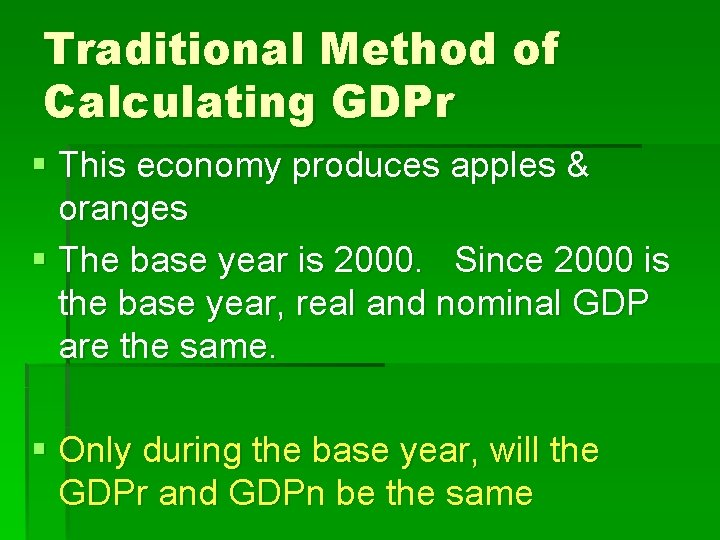Traditional Method of Calculating GDPr § This economy produces apples & oranges § The base year is 2000. Since 2000 is the base year, real and nominal GDP are the same. § Only during the base year, will the GDPr and GDPn be the same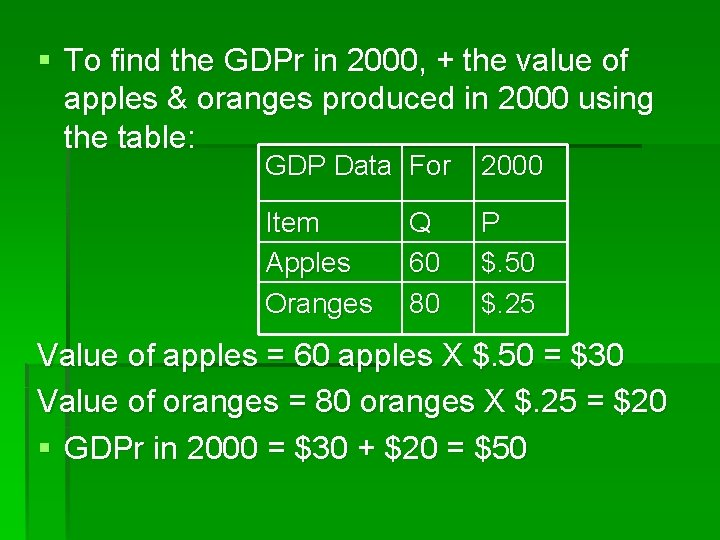§ To find the GDPr in 2000, + the value of apples & oranges produced in 2000 using the table: GDP Data For 2000 Item Apples Oranges P \$. 50 \$. 25 Q 60 80 Value of apples = 60 apples X \$. 50 = \$30 Value of oranges = 80 oranges X \$. 25 = \$20 § GDPr in 2000 = \$30 + \$20 = \$50§ To calculate GDPr in 2006, + the value of apples and oranges using the prices of 2000 GDP Data Item Apples Oranges For Q 160 220 2006 P \$1. 00 \$2. 00 § Value of apples = 160 apples X \$. 50 = \$80 § Value of oranges = 220 oranges X \$. 25 = \$55 § GDPr in 2006 = \$80 + \$55 = \$135 § Nominal would be? § \$6002 purposes of estimating Real GDP § To compare the standard of living over time (based on quantity, not price) § To compare the standard of living among countries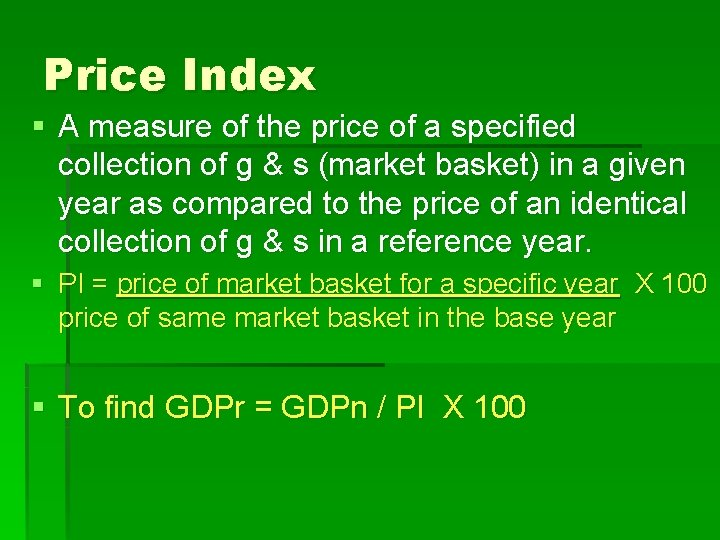Price Index § A measure of the price of a specified collection of g & s (market basket) in a given year as compared to the price of an identical collection of g & s in a reference year. § PI = price of market basket for a specific year X 100 price of same market basket in the base year § To find GDPr = GDPn / PI X 100GDP Deflator § An average of current prices expressed as a percentage of base year prices. § Measures the price level § The average level of prices § GDP deflator = (GDPn / GDPr) X 100 § Example (\$100 / \$80) X 100 = GDP deflator § 1. 25 X 100= 125 § Prices have gone up 25%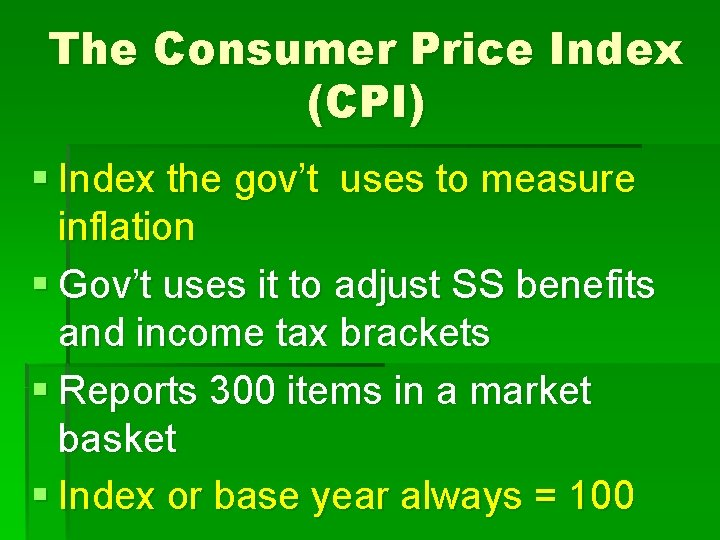The Consumer Price Index (CPI) § Index the gov’t uses to measure inflation § Gov’t uses it to adjust SS benefits and income tax brackets § Reports 300 items in a market basket § Index or base year always = 100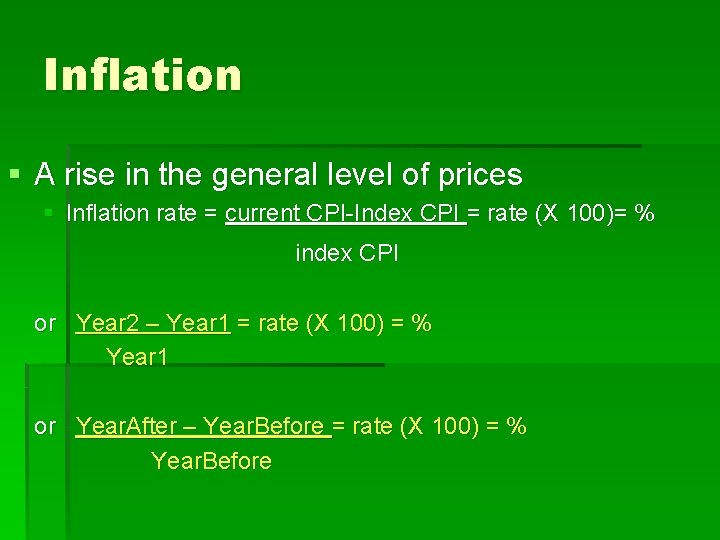Inflation § A rise in the general level of prices § Inflation rate = current CPI-Index CPI = rate (X 100)= % index CPI or Year 2 – Year 1 = rate (X 100) = % Year 1 or Year. After – Year. Before = rate (X 100) = % Year. Before